#### Translations of Conics

Translation of Conics:

The main difference between this part and the upper part is that in this part, the conics have gone via a rigid transformation and been shifted horizontally or vertically.

By substituting each and every x with an x-h and every y with y-k, what utilized to be at the origin is now at point (h, k). All the movement is then relative to that point.

Eccentricity:

Eccentricity is the ratio of distance to the focus divided by the distance to vertex. That is, e = c/a.

All conic section has an eccentricity.

Conic         Eccentricity

Circle         e = 0

Ellipse        0 < e < 1

Parabola     e = 1

Hyperbola    e > 1

Eccentricity is frequently used in astronomy to explain the path of planets, moons or comets which orbit the other object.

Parabola:

The vertex of parabola employed to be at origin. The vertex will now be at point (h, k).

Vertical Axis of Symmetry: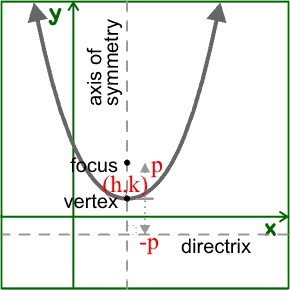Vertical Parabola (x - h)2 = 4 p(y - k)

The vertex is at (h, k).

As the axis of symmetry is vertical, the foci and directrix encompass various y coordinates than the vertex.

As p is the directed distance from the vertex to focus, then the focus will be at (h, k+p)

As the directrix is p units the other side of vertex, the equation of directrix will be equal to y = k-p

The parabola will open up when p is positive and down when p is negative.

Horizontal Axis of Symmetry: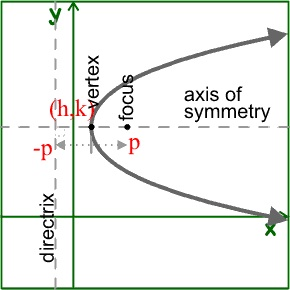Horizontal Parabola (y - k)2 = 4 p (x - h)

The vertex is at (h, k).

As the axis of symmetry is horizontal, the directrix and foci have various x coordinates than vertex.

As p is directed distance from the vertex to focus, the focus will be at (h+p, k)

As the directrix is p units the other side of vertex, then the equation of directrix will be x = h-p

The parabola will open right when p is positive and left when p is negative.

Circle:

(x - h)2 + (y - k)2 = r2

The center of circle used to be at the origin. The center will now be at point (h, k).

Ellipse:

The center of ellipse used to be at origin. The center will now be at point (h, k).

Remember that for an ellipse, the distance from the center to vertices is a, the distance from the center to foci is c, and the distance from center to the end-points of the minor axis is b. The longest of such lengths is the distance to vertices, therefore the

Pythagorean identity for an ellipse is a2 = b2 + c2.

Horizontal Major Axis: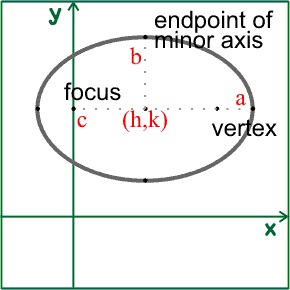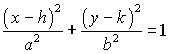As the major axis is horizontal, the foci and vertices will have various x coordinates than the center.

As a is the distance from the center to vertices, the vertices will be at (h+a, k) and (h-a, k)

As c is the distance from the center to foci, then the foci will be at (h+c, k) and (h-c, k).

As b is the distance from the center to endpoints of the minor axis, and the minor axis is vertical, then endpoints of minor axis will be at (h, k+b) and (h, k-b).

Vertical Major Axis: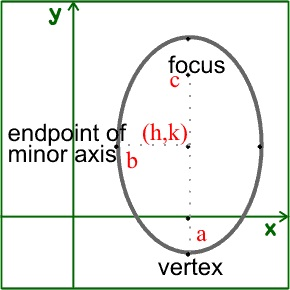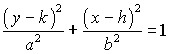As the major axis is vertical, the foci and vertices will have various y-coordinates than the center.

As a is the distance from the center to vertices, then the vertices will be at (h, k+a) and (h, k-a)

As c is the distance from the center to foci, then the foci will be at (h, k+c) and (h, k-c).

As b is the distance from the center to end-points of the minor axis, and the minor axis is horizontal, then the end-points of the minor axis will be at (h+b, k) and (h-b, k).

Hyperbola:

The center of hyperbola utilized to be at the origin. The center will now be at point (h, k).

Remember that for hyperbola, the distance from the center to vertices is a, distance from the center to the foci is c, and the distance from center to the endpoints of conjugate axis is b. The longest of such lengths is the distance to foci, therefore the

Pythagorean identity for a hyperbola is a2 + b2 = c2.

Horizontal Transverse Axis: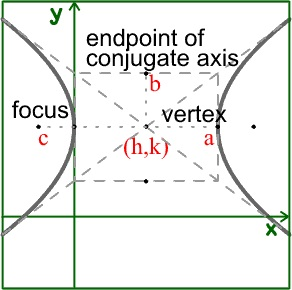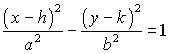Center is at (h, k).

As the transverse axis is horizontal, the foci and vertices will contain different x coordinates than the center.

As a is the distance from the center to vertices, the vertices will be at (h+a, k) and (h-a, k)

As c is the distance from the center to foci, then the foci will be at (h+c, k) and (h-c, k).

As b is the distance from the center to endpoints of the conjugate axis, and the conjugate axis is vertical, then the endpoints of conjugate axis will be at (h, k+b) and (h, k-b).

As the change in y is b and the change in x is a, then the slope of that asymptotes will be ±b/a. The equations of asymptotes will be (y-k) = ± b/a (x-h).

Vertical Transverse Axis: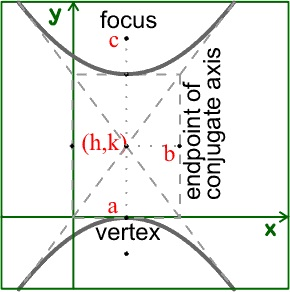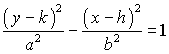The center is at (h, k).

As the transverse axis is vertical, the foci and vertices will contain different y coordinates than the center.

As a is the distance from the center to vertices, then the vertices will be at (h, k+a) and (h, k-a)

As c is the distance from the center to foci, the foci will be at (h, k+c) and (h, k-c).

As b is the distance from the center to end-points of the conjugate axis, and the conjugate axis is horizontal, then end-points of the conjugate axis will be at (h+b, k) and (h-b, k).

As the change in y is a and the change in x is b, then the slope of that asymptotes will be ±a/b. The equations of asymptotes will be (y-k) = ± a/b (x-h).

Latest technology based Algebra Online Tutoring Assistance

Tutors, at the www.tutorsglobe.com, take pledge to provide full satisfaction and assurance in Algebra help via online tutoring. Students are getting 100% satisfaction by online tutors across the globe. Here you can get homework help for Algebra, project ideas and tutorials. We provide email based Algebra help. You can join us to ask queries 24x7 with live, experienced and qualified online tutors specialized in Algebra. Through Online Tutoring, you would be able to complete your homework or assignments at your home. Tutors at the TutorsGlobe are committed to provide the best quality online tutoring assistance for Algebra Homework help and assignment help services. They use their experience, as they have solved thousands of the Algebra assignments, which may help you to solve your complex issues of Algebra. TutorsGlobe assure for the best quality compliance to your homework. Compromise with quality is not in our dictionary. If we feel that we are not able to provide the homework help as per the deadline or given instruction by the student, we refund the money of the student without any delay.